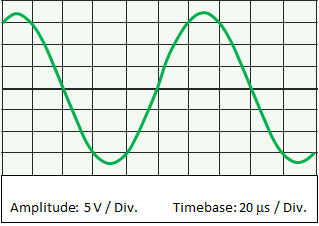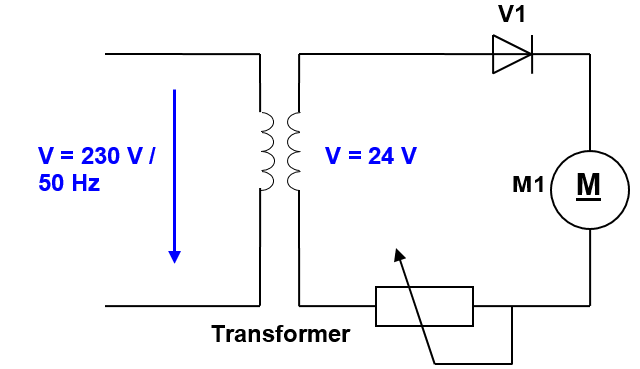(Deutsch)Learnchannel Nachhilfe

Part 1: Multiple Choice -  only one answer is correct!

An alternating voltage has a period time of 20 ms. What is the corresponding frequency?

This voltage curve was measured with an oscilloscope. Which statement is correct?Measured with an oscilloscope

The AC voltage displayed has:

The following information is printed on an LED light: 230 V / 6 W. Which statements about these values are correct?

What is the relation between the RMS value and the peak value of AC?

Part 2: project task

An encoder is flanged to the 24V DC motor and outputs a square wave signal of 4 times the frequency per revolution. How this system works, you can see in the small video clip:

The circuit diagram is shown below:Work order Encoder

1.   For which maximum voltage (peak value!) must the diode V1 be designed if the transformer has an output voltage of 24 V?

The rotational speed is detected by a encoder, which supplies rectangular pulses to the control. The encoder signals at minimum speed and maximum speed are shown below:work order encoder signal

2.   What is the frequency of the encoder signal at low and high speeds.
3.   How long does the motor need for a complete revolution at low and high speed?

=>  Get the solution

The solutions are password-protected. Please send an e-mail to

info@learnchannel-tv.com    ... if you want to get access.